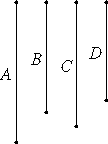# Proposition 31

To find two medial straight lines commensurable in square only, containing a rational rectangle, such that the square on the greater is greater than the square on the less by the square on a straight line commensurable in length with the greater.
X.29

Set out two rational straight lines A and B commensurable in square only such that the square on A, being the greater, is greater than the square on B the less by the square on a straight line commensurable in length with A.Let the square on C equal the rectangle A by B.

X.21

Now the rectangle A by B is medial, therefore the square on C is also medial. Therefore C is also medial.

Let the rectangle C by D equal the square on B.

X.22.Lem

Now the square on B is rational, therefore the rectangle C by D is also rational. And since A is to B as the rectangle A by B is to the square on B, while the square on C equals the rectangle A by B, and the rectangle C by D equals the square on B, therefore A is to B as the square on C is to the rectangle C by D.

But the square on C is to the rectangle C by D as C is to D, therefore A is to B as C is to D.

X.11

But A is commensurable with B in square only, therefore C is also commensurable with D in square only.

X.23,Note

And C is medial, therefore D is also medial.

X.14

Since A is to B as C is to D, and the square on A is greater than the square on B by the square on a straight line commensurable with A, therefore the square on C is greater than the square on D by the square on a straight line commensurable with C.

Therefore two medial straight lines C and D, commensurable in square only and containing a rational rectangle, have been found, and the square on C is greater than the square on D by the square on a straight line commensurable in length with C.

X.30

Similarly also it can be proved that the square on C exceeds the square on D by the square on a straight line incommensurable with C, when the square on A is greater than the square on B by the square on a straight line incommensurable with A.

Q.E.D.

## Guide

This proposition is used in X.34 and X.35.